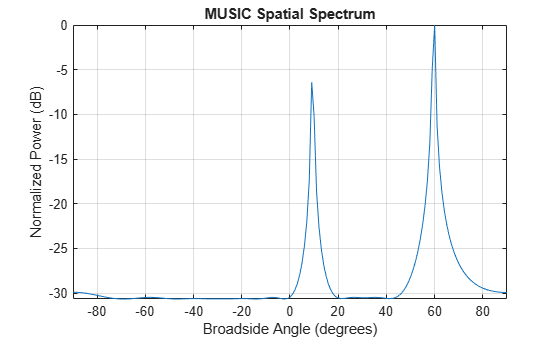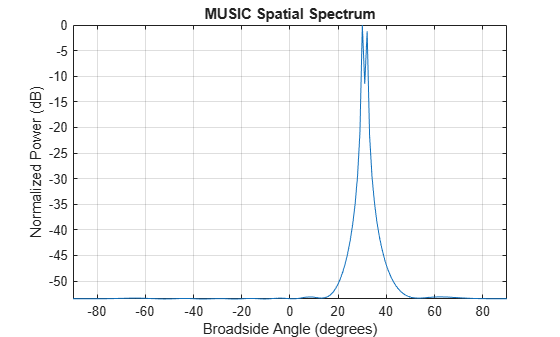# phased.MUSICEstimator

Estimate direction of arrival using narrowband MUSIC algorithm for ULA

## Description

The `phased.MUSICEstimator` System object™ implements the narrowband multiple signal classification (MUSIC) algorithm for uniform linear arrays (ULA). MUSIC is a high-resolution direction-finding algorithm capable of resolving closely-spaced signal sources. The algorithm is based on eigenspace decomposition of the sensor spatial covariance matrix.

To estimate directions of arrival (DOA):

1. Define and set up a `phased.MUSICEstimator` System object. See Construction.

2. Call the `step` method to estimate the DOAs according to the properties of `phased.MUSICEstimator`.

Note

Alternatively, instead of using the `step` method to perform the operation defined by the System object, you can call the object with arguments, as if it were a function. For example, `y = step(obj,x)` and `y = obj(x)` perform equivalent operations.

## Construction

`estimator = phased.MUSICEstimator` creates a MUSIC DOA estimator System object, `estimator`.

`estimator = phased.MUSICEstimator(Name,Value)` creates a System object, `estimator`, with each specified property Name set to the specified Value. You can specify additional name-value pair arguments in any order as (`Name1`,`Value1`,...,`NameN`,`ValueN`).

## Properties

expand all

ULA sensor array, specified as a `phased.ULA` System object. If you do not specify any name-value pair properties for the ULA sensor array, the default properties of the array are used.

Signal propagation speed, specified as a real-valued positive scalar. Units are in meters per second. The default propagation speed is the value returned by `physconst('LightSpeed')`.

Example: `3e8`

Data Types: `single` | `double`

Operating frequency, specified as a positive scalar. Units are in Hz.

Example: `1e9`

Data Types: `single` | `double`

Enable forward-backward averaging, specified as `false` or `true`. Set this property to `true` to use forward-backward averaging to estimate the covariance matrix for sensor arrays with a conjugate symmetric array manifold.

Data Types: `logical`

Broadside scan angles, specified as a real-valued vector. Units are in degrees. Broadside angles are between the search direction and the ULA array axis. The angles lie between –90° and 90°, inclusive. Specify the angles in increasing value.

Example: `[-20:20]`

Data Types: `single` | `double`

Option to enable directions-of-arrival (DOA) output, specified as `false` or `true`. To obtain the DOA of signals, set this property to `true`. The DOAs are returned in the second output argument when the object is executed.

Data Types: `logical`

Source of the number of arriving signals, specified as `'Auto'` or `'Property'`.

• `'Auto'` — The System object estimates the number of arriving signals using the method specified in the `NumSignalsMethod` property.

• `'Property'` — Specify the number of arriving signals using the `NumSignals` property.

Data Types: `char`

Method used to estimate the number of arriving signals, specified as `'AIC'` or `'MDL'`.

• `'AIC'` — Akaike Information Criterion

• `'MDL'` — Minimum Description Length criterion

#### Dependencies

To enable this property, set `NumSignalsSource` to `'Auto'`.

Data Types: `char`

Number of arriving signals for DOA estimation, specified as a positive integer.

Example: `3`

#### Dependencies

To enable this property, set `NumSignalsSource` to `'Property'`.

Data Types: `single` | `double`

Option to enable spatial smoothing, specified as a nonnegative integer. Use spatial smoothing to compute the arrival directions of coherent signals. A value of zero specifies no spatial smoothing. A positive value represents the number of subarrays used to compute the smoothed (averaged) source covariance matrix. Each increment in this value lets you handle one additional coherent source, but reduces the effective number of array elements by one. The length of the smoothing aperture, L, depends on the array length, M, and the averaging number, K, by L = M – K + 1. The maximum value of K is M – 2.

Example: `5`

Data Types: `double`

## Methods

 plotSpectrum Plot MUSIC spectrum reset Reset states of System object step Estimate direction of arrival using MUSIC
Common to All System Objects
`release`

Allow System object property value changes

## Examples

collapse all

Estimate the DOAs of two signals received by a standard 10-element ULA having an element spacing of 1 meter. Then plot the MUSIC spectrum.

Create the ULA array. The antenna operating frequency is 150 MHz.

```fc = 150.0e6; array = phased.ULA('NumElements',10,'ElementSpacing',1.0);```

Create the arriving signals at the ULA. The true direction of arrival of the first signal is 10° in azimuth and 20° in elevation. The direction of the second signal is 60° in azimuth and -5° in elevation.

```fs = 8000.0; t = (0:1/fs:1).'; sig1 = cos(2*pi*t*300.0); sig2 = cos(2*pi*t*400.0); sig = collectPlaneWave(array,[sig1 sig2],[10 20; 60 -5]',fc); noise = 0.1*(randn(size(sig)) + 1i*randn(size(sig)));```

Estimate the DOAs.

```estimator = phased.MUSICEstimator('SensorArray',array,... 'OperatingFrequency',fc,... 'DOAOutputPort',true,'NumSignalsSource','Property',... 'NumSignals',2); [y,doas] = estimator(sig + noise); doas = broadside2az(sort(doas),[20 -5])```
```doas = 1×2 9.5829 60.3813 ```

Plot the MUSIC spectrum.

`plotSpectrum(estimator,'NormalizeResponse',true)`First, estimate the DOAs of two signals received by a standard 10-element ULA having an element spacing of one-half wavelength.Then, plot the spatial spectrum.

The antenna operating frequency is 150 MHz. The arrival directions of the two signals are separated by 2°. The direction of the first signal is 30° azimuth and 0° elevation. The direction of the second signal is 32° azimuth and 0° elevation. Estimate the number of signals using the Minimum Description Length (MDL) criterion.

Create the signals arriving at the ULA.

```fs = 8000; t = (0:1/fs:1).'; f1 = 300.0; f2 = 600.0; sig1 = cos(2*pi*t*f1); sig2 = cos(2*pi*t*f2); fc = 150.0e6; c = physconst('LightSpeed'); lam = c/fc; array = phased.ULA('NumElements',10,'ElementSpacing',0.5*lam); sig = collectPlaneWave(array,[sig1 sig2],[30 0; 32 0]',fc); noise = 0.1*(randn(size(sig)) + 1i*randn(size(sig)));```

Estimate the DOAs.

```estimator = phased.MUSICEstimator('SensorArray',array,... 'OperatingFrequency',fc,'DOAOutputPort',true,... 'NumSignalsSource','Auto','NumSignalsMethod','MDL'); [y,doas] = estimator(sig + noise); doas = broadside2az(sort(doas),[0 0])```
```doas = 1×2 30.0000 32.0000 ```

Plot the MUSIC spectrum.

`plotSpectrum(estimator,'NormalizeResponse',true)`expand all

## References

 Van Trees, H. L. Optimum Array Processing. New York: Wiley-Interscience, 2002.

## Version History

Introduced in R2016b